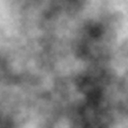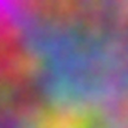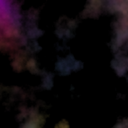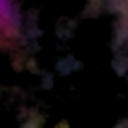# Creating Nebulae

This article describes the algorithm I used for creating the nebulae. I have no idea if someone has invented this before and there are many other ways (probably better) of doing the trick. Anyway, I hope you find this article useful.

You can find example pictures from the Screenshots page.

The main component in creating the nebulae is Perlin noise:(you can click the images to view them in larger size)

You can find more info about it and a few ready implemetations here, here, here, here, here, here and here. (This is not a Perlin noise tutorial.)

We will also need an exponent filter, which is pretty simple, yet very useful (can be also used, for example, to create star images, flares or clouds).

Pseudo code follows:

Function ExpFilter(value, cover, sharpness)
c = value - (255 - cover)  //reduce coverage
If c < 0 Then c = 0            // prevent from going negative
value = 255 - ((sharpness^c)*255) // do the magic
Return value                     // return result
End Function

The code assumes that value to filter is between 0 and 255 as is cover (the bigger cover is the brighter the outcome will be). Sharpness is a float, a little under 1 e.g. 0.99 and it tells how hard the edges of the "clouds" are. The return value is also between 0 and 255.

So by putting some Perlin noise through an exponent filter we get something that resembles clouds:Notice how the most bright areas in the original image are still present in the filtered one.

But we didn't want clouds, so lets try to add some color. First we just create something colorful. This step is fairly simple, let's just take three differently seeded Perlin noise functions and assign them as the red, green and blue color values. The output:I used noise functions that change in a relatively lazy way (high octaves), and by changing that, one can adjust how much there is different color in one nebula (or cloud). Then we just combine the cloud like alphamap with this colorful mess by an operation called multiply (a sort of alpha blending would also be possible):

blendMultiply(a,b)
((a * b) Shr 8)

Where a is the color value and b is the value from the "cloud" map and Shr stands for Shift Right, which means shifting the bits of the result right 8 places, efectively dividing it by 2 eight times. This is done to each of the three color values (red,green and blue) of each pixel.

And behold, beautiful nebulae are born!Lets add a few stars to make the black look less boring (this is just a quick bonus :) ).If you wish, you can take a peek at the source code (in FreeBASIC) I used for creating the images for this tutorial here. (Note that it doesn't include the libNoise which contains an implementation of Perlin noise and an exponent filter function similar to that I represented here.)

That's it. Of course in my game the pixels are represented by ascii character 219 (█) and stars are asterisks, but that's quite irrelevant. If you have any questions or comments (and please do!), post them to the forums.

Update: libNoise, along with the tutorial code can be downloaded from github.